## An electron is accelerated by a uniform electric field (1000 V/m) pointing vertically upward. 1) Use Newton’s laws to determine the electron

Question

An electron is accelerated by a uniform electric field (1000 V/m) pointing vertically upward. 1) Use Newton’s laws to determine the electron’s velocity after it moves 0.10 cm from rest. 2) Find the speed using the energy method. That is, consider the electric potential energy change and conversion to kinetic

in progress 0
5 months 2021-09-05T00:14:23+00:00 1 Answers 3 views 0

The velocity of electron is 592999.45 m/s.

Explanation:

Given that,

Electric field = 1000 V/m

Distance = 0.10 cm

(a).We need to calculate the velocity of electron

Using newton’s law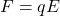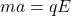…(I)

Now, Using equation of motion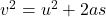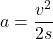Put the value of a in equation (I)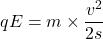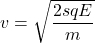Put the value into the formula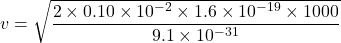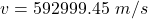(b). We need to calculate the velocity of electron

Using work theorem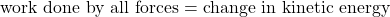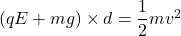Here, weight of electron is very less compare to electric field

So, neglect to weight of electron.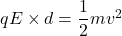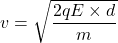Put the value into the formula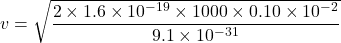Hence, The velocity of electron is 592999.45 m/s.